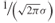# A.1 Probability Distribution Functions

In this appendix we give the probability density functions (PDFs) and the basic properties of the most commonly used statistical distributions in the characterization of the fading envelope of the received signal. These include the Rayleigh, the Rician, the Nakagami m-distribution, Weibull, the log-normal, the Suzuki and the chi-square distributions. In addition, we give the Gaussian distribution used to represent thermal noise, which is also used in the generation of Rayleigh distribution.

# A.2 The Gaussian (Normal) Distribution

A random variable r is said to be normally distributed with mean μ and variance σ2 if its probability density function is:

A.1Since the normal or Gaussian distribution is fully characterized by its mean and variance or standard deviation σ it is usually represented by:

A.2The Gaussian distribution has a bell-shaped curve with its maximum value ofoccurring at the mean value μ. Figure A.1 illustrates the distribution for different values of the mean and standard deviation σ where it can be seen that the standard deviation is related to the dispersion around the mean.

Figure A.1 Normal distribution for various ...

Get Radio Propagation Measurement and Channel Modelling now with the O’Reilly learning platform.

O’Reilly members experience live online training, plus books, videos, and digital content from nearly 200 publishers.